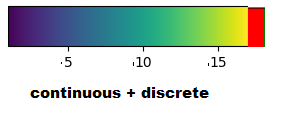# vtkScalarBarActor usage

Hello Everyone,

I’m using `vedo` to create a network with nodes and edges and color the nodes using the scalar values stored in an array. From what I understand, `vedo` uses `vtkScalarBarActor` to associate the scalar values to colors.
Code:

``````import networkx as nx
from vedo import *

G = nx.gnm_random_graph(n=10, m=15, seed=1)
nxpos = nx.spring_layout(G)
nxpts = [nxpos[pt] for pt in sorted(nxpos)]

nx_lines = []
for i, j in G.edges():
p1 = nxpos[i].tolist() +   # add z-coord
p2 = nxpos[j].tolist() + 
nx_lines.append([p1, p2])

nx_pts = Points(nxpts, r=12)
nx_edg = Lines(nx_lines).lw(2)

# node values
vals = [100, .80, .10, .79, .70, .60, .75, .78, .65, .90]
show(nx_pts, nx_edg, nx_pts.labels('id'), interactive=True, bg='black', title='plot')
``````

I’m facing a problem when there are outliers in the scalar values.

` vals = [100, .80, .10, .79, .70, .60, .75, .78, .65, .90]` in here there is one outlier and due to this the rest of the values are assigned a single color. I would like to ask for suggestions on how to avoid the above and create a scalarbar like the followingThe red discrete patch in the bottom representation is for the outliers (i.e 100 in vals = [100, .80, .10, .79, .70, .60, .75, .78, .65, .90])

More details on the same issue can be found here

Hello,

I suggest you to study or find examples using `vtkColorTransferFunction` class: https://vtk.org/doc/nightly/html/classvtkColorTransferFunction.html. You programmaticaly build it with calls to its `addRGBSegment()` method. First, make sure the transfer function is clear by making a prior call to `RemoveAllPoints()`. For the valid continous values you do something like `addRGBSegment(0.7, r1, g1, b1, 0.8, r2, g2, b2)`. For the outlier code (e.g. 100) you do like `addRGBSegment(99.5, r, g, b, 100.5, r, g, b)`. Notice the usage of the same RGB values for the range that includes the 100 value.

After your transfer function is defined, you build a LUT with this recipe (in C++, sorry):

``````    //create the color table object
vtkSmartPointer<vtkLookupTable> lut = vtkSmartPointer<vtkLookupTable>::New();
lut->SetTableRange(0.0, 100.5);
lut->SetNumberOfTableValues(64);
for(size_t i = 0; i < tableSize; ++i)
{
double *rgb;
double value = /* lookup/compute value from i and 0.0-1.0; 100 values */;
rgb = transferFunction->GetColor( value ); //value can be 0.0-1.0 and 100
lut->SetTableValue(i, rgb, rgb, rgb);
}
lut->SetRampToLinear(); //you may play with other interpolation methods
lut->Build();
``````

I hope this helps.

regards,

Paulo

1 Like

Hi Paulo, Thank you so much for the details.